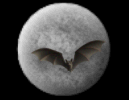God & Science Forum Message Forums: Atm · Astrophotography · Blackholes · Blackholes2 · CCD · Celestron · Domes · Education Eyepieces · Meade · Misc. · God and Science · SETI · Software · UFO · XEphemBe the first pioneers to continue the Astronomy Discussions at our new Astronomy meeting place...The Space and Astronomy Agora To Be More Exact Forum List | Follow Ups | Post Message | Back to Thread Topics | In Response ToPosted by Tarvo on November 15, 2003 15:14:51 UTC

Any state machine like computer what implements continuous process needs a loop (like a loop in algorithm) in Gödel's formal system because such state machine cannot be implemented as a function of time or by calculating just one state. Most changing structures can only be implemented in the computer, equations cannot model them other than iterative equations what implement computer or similar state machine. It is that for saying anything it's necessary to partly refute the Gödel's theorem, in spite that the argument of non-computability is obviously wrong considering a huge number of programs written in so many years, and not yet anybody didn't find a single case when something couldn't be programmed because of non-computability. But I still think that Gödel's theorem is useful to show the limits of (non-iterative) equations.

Gödel's original paper: http://home.ddc.net/ygg/etext/godel/godel3.htm

This is how the reference of series of basic signs is calculated "Furthermore, variables of type n are given numbers of the form pn (where p is a prime number > 13). Hence, to every finite series of basic signs (and so also to every formula) there corresponds, one-to-one, a finite series of natural numbers. These finite series of natural numbers we now map (again in one-to-one correspondence) on to natural numbers, by letting the number 2n1, 3n2 … pknk correspond to the series n1, n2, … nk, where pk denotes the k-th prime number in order of magnitude. A natural number is thereby assigned in one-to-one correspondence, not only to every basic sign, but also to every finite series of such signs. We denote by F(a) the number corresponding to the basic sign or series of basic signs a." Now to have a loop, we supposed to have such reference in the formula, but then the reference to the formula would be bigger than that reference and there's supposed to be no way how we can write such loop in Gödel's formal system.